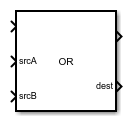# OR

• Library:
•## Description

The OR block implements the `OR` ladder logic instruction. When the rung conditions are true, the block performs bitwise OR operation on the values at source A with the values at source B. The result of this operation is available at the destination port (`dest`).

## Ports

### Input

expand all

Controls the execution of the block. EnableIn reflects the rung state preceding the block. If the rung state preceding the block is false, EnableIn is false, the block does not execute and the outputs are not updated.

The first input signal to the bitwise OR operation. If the datatype is `single` (`REAL` – ladder logic equivalent), the input value is converted to `int32` (`DINT` – ladder logic equivalent). `int8, int16` (`SINT,INT` – ladder logic equivalent) datatypes are converted to `int32` (`DINT` – ladder logic equivalent) by filling the upper bits with 0s.

Data Types: `int8` | `int16` | `int32` | `single`

The second input signal to the bitwise OR operation. If the datatype is `single` (`REAL` – ladder logic equivalent), the input value is converted to `int32` (`DINT` – ladder logic equivalent). `int8, int16` (`SINT,INT` – ladder logic equivalent) datatypes are converted to `int32` (`DINT` – ladder logic equivalent) by filling the upper bits with 0s.

Data Types: `int8` | `int16` | `int32` | `single`

### Output

expand all

By default, EnableOut follows the state of EnableIn. If the EnableIn input to the block is false, the logic implemented by the block is not executed and EnableOut signal is set to false.

Output signal resulting from the bitwise OR operation. If the datatype is `single` (`REAL` – ladder logic equivalent), the resultant `int32` (`DINT` – ladder logic equivalent) is converted to `REAL` (`single` – ladder logic equivalent).

Data Types: `int8` | `int16` | `int32` | `single`